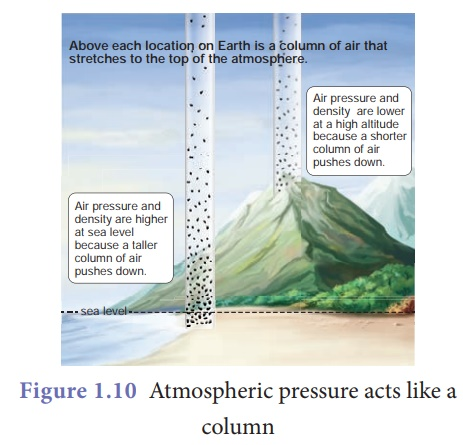Home | | Science 9th std | Atmospheric pressure

# Atmospheric pressure

Earth is surrounded by a layer of air up to certain height (nearly 300 km) and this layer of air around the earth is called atmosphere of the earth. Since air occupies space and has weight, it also exerts pressure. This pressure is called atmospheric pressure. The atmospheric pressure we normally refer is the air pressure at sea level.

Atmospheric pressure

Earth is surrounded by a layer of air up to certain height (nearly 300 km) and this layer of air around the earth is called atmosphere of the earth. Since air occupies space and has weight, it also exerts pressure (Fig. 1.9). This pressure is called atmospheric pressure. The atmospheric pressure we normally refer is the air pressure at sea level.Figure 1.10 shows that air gets 'thinner' with increasing altitude. Hence, the atmospheric pressure decreases as we go up in mountains. On the other hand air gets heavier as we go down below sea level like mines. Table 1.1 gives the value of atmospheric pressure at some places above and below sea level.## 1. Measurement ofatmospheric pressure

The instrument used to measure atmospheric pressure is called barometer. A mercury barometer, first designed by an Italian Physicist Torricelli, consists of a long glass tube (closed at one end, open at the other) filled with mercury and turned upside down into a container of mercury. This is done by closing the open end of the mercury filled tube with the thumb and then opening it after immersing it in to a trough of mercury (Fig. 1.11). The barometer works by balancing the mercury in the glass tube against the outside air pressure.If the air pressure increases, it pushes more of the mercury up into the tub and if the air pressure decreases, more of the mercury drains from the tube. As there is no air trapped in the space between mercury and the closed end, there is vacuum in that space. Vacuum cannot exert any pressure. So the level of mercury in the tube provides a precise measure of air pressure which is called atmospheric pressure. This type of instrument can be used in a lab or weather station.On a typical day at sea level, the height of the mercury column is 760 mm. Let us calculate the pressure due to the mercury column of 760 mm which is equal to the atmospheric pressure. The density of mercury is 13600 kg m–3.

Pressure, P = hρg

= (760 x 10–3m) x (13600 kgm–3) x (9.8 ms–2)

= 1.013 x 105 Pa.

This pressure is called one atmospheric pressure (atm). There is also another unit called (bar) that is also used to express such high values of pressure.

1 atm = 1.013 × 105 Pa.

1 bar = 1 × 105 Pa.

Hence, 1 atm = 1.013 bar.

Expressing the value in kilopascal gives 101.3 k Pa. This means that, on each 1 m2 of surface, the force acting is 1.013 k N.

## 2. Types of barometers

As the mercury is not in a closed vessel in the mercury barometer, moving the instrument without spilling the mercury is difficult. Hence, we have other sophisticated instruments which are handy. They also work on the same principle like a mercury barometer but instead of mercury they use diaphragms and other precise components which respond for variation in atmospheric pressure. Table 1.2 shows some of the barometers used frequently.

### Example 1.3

A mercury barometer in a physics laboratory shows a 732 mm vertical column of mercury. Calculate the atmospheric pressure in pascal. [Given density of mercury, ρ = 1.36 × 104 kg m–3, g = 9.8 m s–2]

### Solution:

Atmospheric pressure in the laboratory,

P = hρg = 732 × 10–3 × 1.36 × 104 × 9.8

= 9.76 × 104 Pa (or) 0.976 × 105 Pa## 3. Gauge pressure andabsolute pressure

Our daily activities are happening in the atmospheric pressure. We are so used to it that we do not even realise. When tyre pressure and blood pressure are measured using instruments (gauges) they show the pressure over the atmospheric pressure. Hence, absolute pressure is zero-referenced against a perfect vacuum and gauge pressure is zero-referenced against atmospheric pressure.

For pressures higher than atmospheric pressure, absolute pressure = atmospheric pressure + gauge pressure

For pressures lower than atmospheric pressure, absolute pressure = atmospheric pressure – gauge pressure

### Example 1.4

Find the absolute pressure on a scuba diver (deep sea diver) when the diver is 12 metres below the surface of the ocean. Assume standard atmospheric conditions. [Take density of water as 1030 kg m–3, g = 9.8 m s–2]

### Solution:

Pressure due to sea water, Pwater = h ρg

= (12 m) × (1.03 ×103 kgm–3) × (9.8 m s–2)

= 1.21 × 105 Pa

Pabsolute = Patmosphere + Pwater

= (1.01 × 105) + (1.21 × 105)

Pabsolute = 2.22 × 105 Pa

This is more than twice the atmospheric pressure. Parts of our body, especially blood vessels and soft tissues cannot withstand such high pressure. Hence, scuba divers always wear special suits and equipment to protect them (Fig. 1.12).### Activity 5

Press a good quality rubber sucker hard on a plane smooth surface. It sticks to the surface. Now pull it off the surface. When you press the sucker, most of the air between its cup and the plane surface escapes out. The sucker sticks to the plane surface since the pressure due to the atmosphere pushes on it. The sucker can be removed off the plane surface by applying a large external force that overcomes the atmospheric pressure. By this principle only, lizards and monitor lizards (udumbu) are able to get good grip over surfaces.Study Material, Lecturing Notes, Assignment, Reference, Wiki description explanation, brief detail
9th Science : Fluids : Atmospheric pressure |